Courses

# Test: Conformational Analysis- 2

## 14 Questions MCQ Test Chemistry Class 11 | Test: Conformational Analysis- 2

Description
This mock test of Test: Conformational Analysis- 2 for JEE helps you for every JEE entrance exam. This contains 14 Multiple Choice Questions for JEE Test: Conformational Analysis- 2 (mcq) to study with solutions a complete question bank. The solved questions answers in this Test: Conformational Analysis- 2 quiz give you a good mix of easy questions and tough questions. JEE students definitely take this Test: Conformational Analysis- 2 exercise for a better result in the exam. You can find other Test: Conformational Analysis- 2 extra questions, long questions & short questions for JEE on EduRev as well by searching above.
*Multiple options can be correct
QUESTION: 1

### Direction (Q. Nos. 1- 8) This section contains 8 multiple choice questions. Each question has four choices (a), (b), (c) and (d), out of which ONE or  MORE THANT ONE  is correct.   Q. Among the butane conformers, when viewed about C2-C3 bond, which occur at energy minima on a graph of potential energy versus dihedral angle?

Solution:
*Multiple options can be correct
QUESTION: 2

### The correct statement regarding cyclopropane is/are

Solution:

The correct answers are Options A and C.
In cyclopropane the angle strain is maximum. Hence, it is a highly strained molecule and consequently most unstable.In cyclopropane, the adjacent CH2 groups are also eclipsed. Unlike in ethane, this strain cannot be relieved through rotation (the ring is too rigid).  In other words, the CH2 groups are locked in the eclipsed conformation, which results in torsional strain – much like a propeller that has been wound up but held in position.

*Multiple options can be correct
QUESTION: 3

### Select the correct statement(s) concerning conformers of ethane and hexachloroethane?

Solution:
*Multiple options can be correct
QUESTION: 4

The correct statement concerning various conformers of 2-fluoroethanol is/are

Solution:
*Multiple options can be correct
QUESTION: 5

Consider the various conformers possible for meso form and enantiomeric 1,2-dibromo-1,2-dichloroethane and select the correct statement(s).

Solution:
*Multiple options can be correct
QUESTION: 6

Select the correct statement(s) regarding conformers of 1,2-dimethyl cyclohexane.

Solution:
*Multiple options can be correct
QUESTION: 7

The correct statement regarding conformation in butane is/are

Solution:
*Multiple options can be correct
QUESTION: 8

the correct statement(s) concerning the following Newmann's projection is/are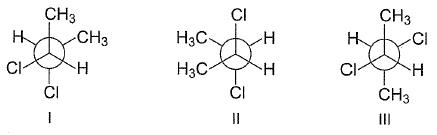Solution:
QUESTION: 9

Direction (Q. Nos. 9-14) This section contains 2 paragraph, wach describing  theory, experiments, data etc. three Questions related to paragraph have been  given.Each question have only one correct answer among the four given  ptions  (a),(b),(c),(d)

Passage I

The two major contributors of conformers of 1,2-dichloroethane are anti and gauche. At 32°C in gas phase, the measured dipole moment of 1,2-dichloroethane is 1.12 D. The dipole moment of a mixture of X and Y is given by the relationship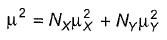Here, N = mole fraction of each kind of molecule. From bond moment measurement, it has been estimated that gauche conformer of 1,2-dichloroethane should have a dipole moment of about 3.2 D.

Q.

What per cent of conformers of 1,2-dichloroethane is anti, at 32°C?

Solution:
QUESTION: 10

Passage I

The two major contributors of conformers of 1,2-dichloroethane are anti and gauche. At 32°C in gas phase, the measured dipole moment of 1,2-dichloroethane is 1.12 D. The dipole moment of a mixture of X and Y is given by the relationshipHere, N = mole fraction of each kind of molecule. From bond moment measurement, it has been estimated that gauche conformer of 1,2-dichloroethane should have a dipole moment of about 3.2 D.

Q.
What is true about percentage of gauche conformer?

Solution:
QUESTION: 11

Passage I

The two major contributors of conformers of 1,2-dichloroethane are anti and gauche. At 32°C in gas phase, the measured dipole moment of 1,2-dichloroethane is 1.12 D. The dipole moment of a mixture of X and Y is given by the relationshipHere, N = mole fraction of each kind of molecule. From bond moment measurement, it has been estimated that gauche conformer of 1,2-dichloroethane should have a dipole moment of about 3.2 D.

Q.

What happens if temperature if increased to 52°C?

Solution:
QUESTION: 12

Passage II

Among conformers, the barrier to rotation originate mainly from torsional strain when atoms or groups eclipse one another. Followings are the costs of energy required to cross the rotational barrier for the indicated pair of groups eclipsing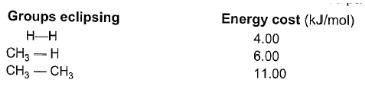Q.

What is the energy barrier to rotation in neopentane ?

Solution:
QUESTION: 13

Passage II

Among conformers, the barrier to rotation originate mainly from torsional strain when atoms or groups eclipse one another. Followings are the costs of energy required to cross the rotational barrier for the indicated pair of groups eclipsingQ.

What is the energy barrier to rotation in 2-methyl propane ?

Solution:

The correct answer is Option D.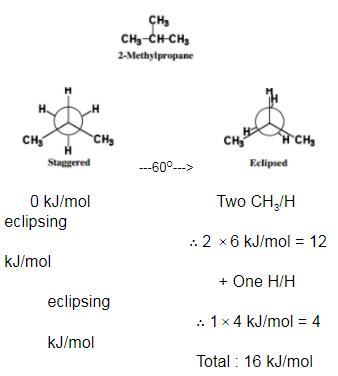QUESTION: 14

Passage II

Among conformers, the barrier to rotation originate mainly from torsional strain when atoms or groups eclipse one another. Followings are the costs of energy required to cross the rotational barrier for the indicated pair of groups eclipsing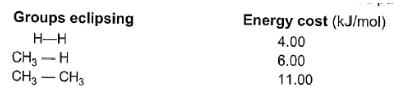Q.

What is the energy difference between two eclipsed conformers of butane when viewed between C2-C3 bond ?

Solution: